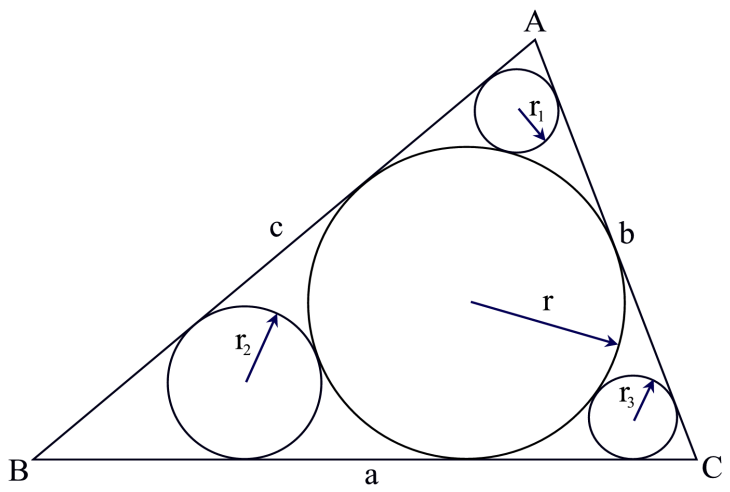시간 제한메모리 제한제출정답맞힌 사람정답 비율
1 초 128 MB552100.000%

## 문제

In the figure below you can see triangle ABC and its in-circle (Circle that touches all the sides of a triangle internally). The radius of this in circle is r. Three other circles are drawn. Each of them touches two sides of this triangle and the in circle of ABC. The radiuses of these circles are r1, r2 and r3.Given the values of r, r1, r2 and r3 you will have to find the area of triangle ABC.

## 입력

The input file can contain up to 1000 lines of inputs. Each line contains four positive floatingpoint numbers which denotes the values of r, r1, r2 and r3 respectively.

Input is terminated by a line containing four negative integers.

## 출력

For each line of input produce one line of output. This line contains serial of output followed by a floating-point number which denotes the area of triangle ABC. This floating-point number may have two digits after the decimal point. You can assume that for the given values of r, r1, r2 and r3 it will always be possible to construct a triangle ABC. If required you can assume that pi = 3.141592653589793 and also use double precision floating-point numbers for floating-point calculations. You can assume that there will be no such input for which small precision errors will cause difference in printed output. Look at the output for sample input for details.

## 예제 입력 1

49.1958415692 5.3025839959 20.7869367050 31.8019699761
186.6830516757 71.9474500429 84.8796672233
37.6219288070
-1 -1 -1 -1


## 예제 출력 1

Case 1: 18237.14
Case 2: 195777.32